# #778. 【NOIP2022】喵了个喵

• 选择一个栈，将牌堆顶上的卡牌放入栈的顶部。如果这么操作后，这个栈最上方的两张牌有相同的图案，则会自动将这两张牌消去。
• 选择两个不同的栈，如果这两个栈栈的卡牌有相同的图案，则可以将这两张牌消去，原来在栈底上方的卡牌会成为新的栈底。如果不同，则什么也不会做。

### 样例一

#### input

1
2 4 2
1 2 1 2

#### output

5
1 1
1 1
1 2
2 1 2
1 1

#### explanation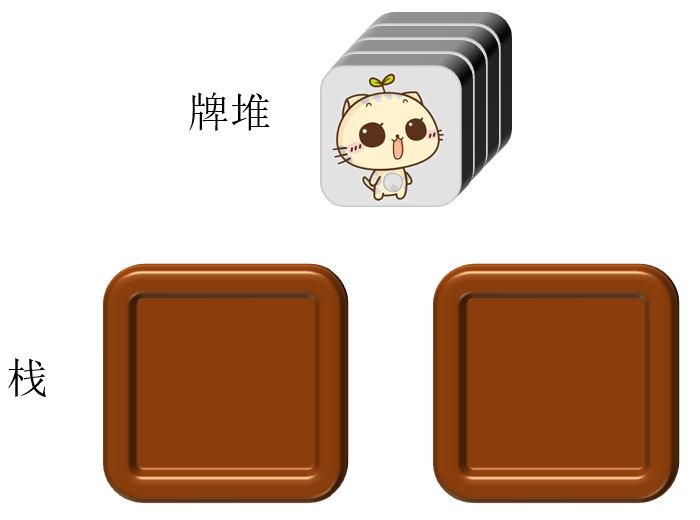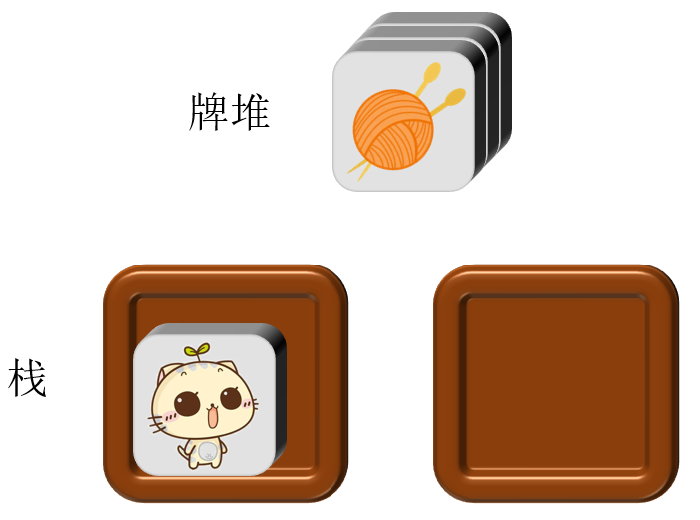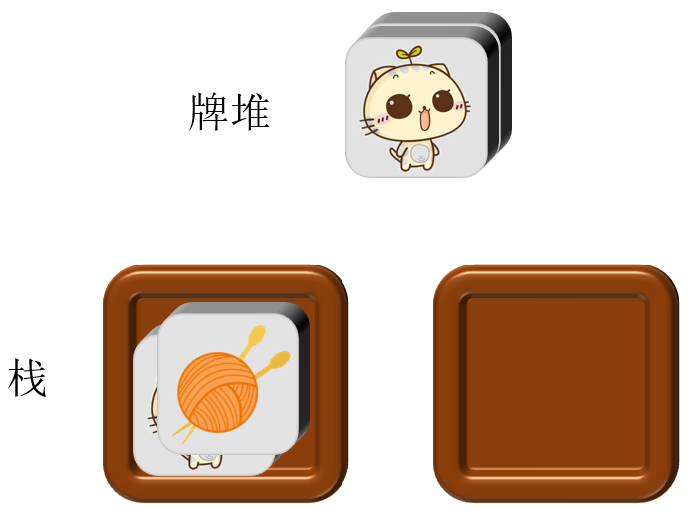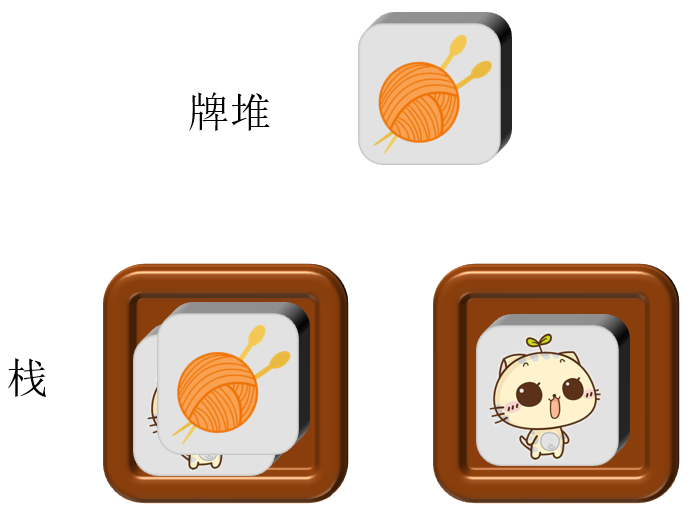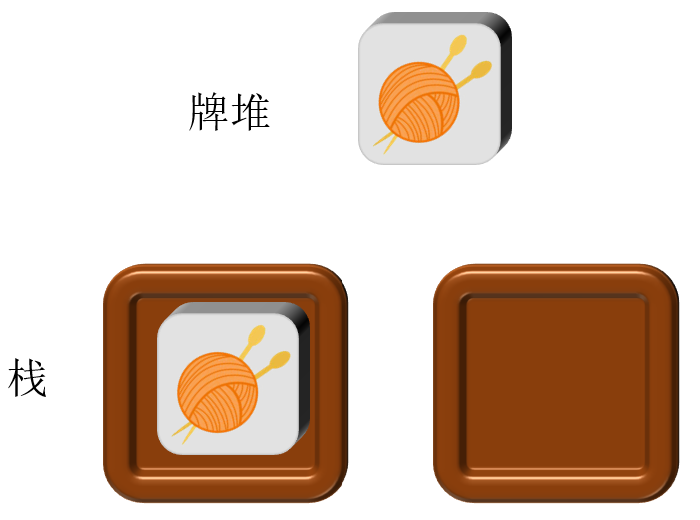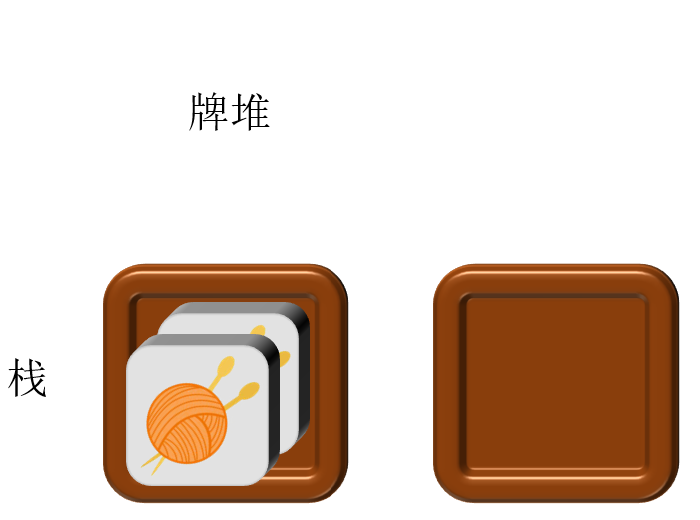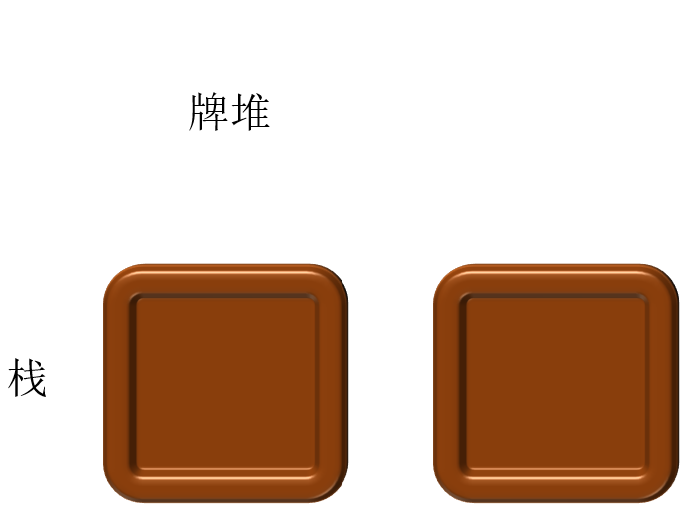### 子任务

$1\sim 3$ $1001$ $\leq 300$ $2n-2$ 无限制
$4\sim 6$ $1002$ $=2$ $2n-1$
$7\sim 10$ $3$ $=3$ $14$
$11\sim 14$ $1004$ 无限制
$15\sim 20$ $1005$ $\leq 300$